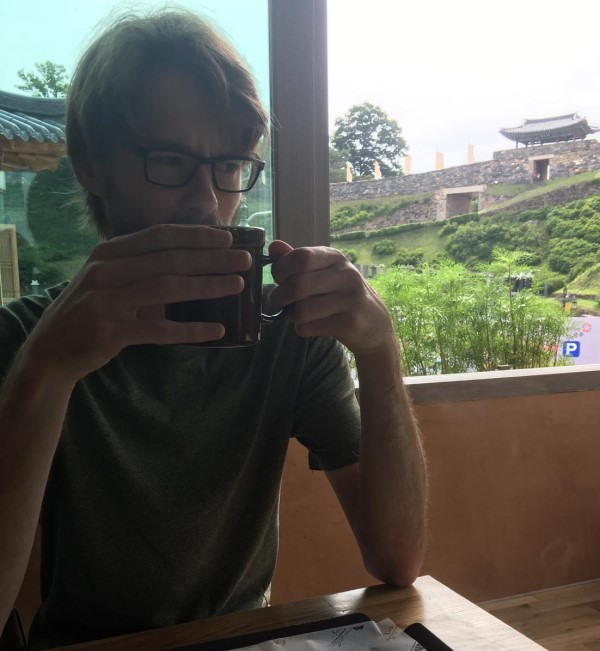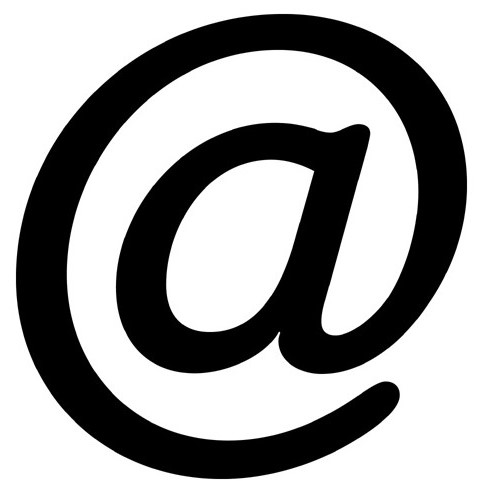# Joost Nuiten### Contact

joost.nuitenmath.univ-toulouse.fr

Institut de Mathématiques de Toulouse (IMT)
Université Paul Sabatier
118 route de Narbonne
F-31062 Toulouse Cedex 9, France

IMT
Université Paul Sabatier
118 route de Narbonne
F-31062 Toulouse Cedex 9, France

I am a maître de conférences at the Institut de Mathématiques de Toulouse, Université Paul Sabatier.

Previously, I was a postdoc in the group of Damien Calaque at the Université de Montpellier, as part of the ERC project `Derived Symplectic Geometry and Applications'. Before that, I was a postdoc at the MPIM Bonn and a PhD student with Ieke Moerdijk at the Universiteit Utrecht.

I am interested in homotopy theory and derived algebraic geometry, in particular deformation theory and its relation to Lie algebroids. My work also tends to involve the theory of operads and algebras over them.

## (Pre)publications

• Two-variable fibrations, factorisation systems and ∞-categories of spans (with R. Haugseng, F. Hebestreit, S. Linskens). pdf, arXiv.

Preprint, 2022.

Abstract: We prove a universal property for ∞-categories of spans in the generality of Barwick's adequate triples, explicitly describe the cocartesian fibration corresponding to the span functor, and show that the latter restricts to a self-equivalence on the class of orthogonal adequate triples, which we introduce for this purpose.

• On straightening for Segal spaces. pdf, arXiv.

Preprint, 2021.

Abstract: We study cocartesian fibrations between (∞, d)-categories in terms of higher Segal spaces and prove a version of straightening for them. This follows from a repeated application of an explicit combinatorial result, relating two types of fibrations between double categories.

• PD operads and explicit partition Lie algebras (with L. Brantner, R. Campos). pdf, arXiv.

Preprint, 2021.

Abstract: Infinitesimal deformations are governed by partition Lie algebras. In characteristic 0, these higher categorical structures are modelled by differential graded Lie algebras, but in characteristic p, they are more subtle. We give explicit models for partition Lie algebras over general coherent rings, both in the setting of spectral and derived algebraic geometry. For the spectral case, we refine operadic Koszul duality to a functor from operads to divided power operads, by taking refined linear duals of Σn-representations. The derived case requires a further refinement of Koszul duality to a more genuine setting.

• Lie algebroids are curved Lie algebras (with D. Calaque, R. Campos). pdf, arXiv.

Preprint, 2021.

Abstract: We show that there is an equivalence of ∞-categories between Lie algebroids and certain kinds of curved Lie algebras. For this we develop a method to study the ∞- category of curved Lie algebras using the homotopy theory of algebras over a complete operad.

• On k-invariants for (∞,n)-categories (with Y. Harpaz, M. Prasma). pdf, arXiv.

Preprint, 2020.

Abstract: Every (∞,n)-category can be approximated by its tower of homotopy (m,n)-categories. In this paper, we prove that the successive stages of this tower are classified by k-invariants, analogously to the classical Postnikov tower for spaces. Our proof relies on an abstract analysis of Postnikov-type towers equipped with k-invariants, and also yields a construction of k-invariants for algebras over ∞-operads and enriched ∞-categories.

• Lax monoidal adjunctions, two-variable fibrations and the calculus of mates (with R. Haugseng, F. Hebestreit, S. Linskens). pdf, arXiv.

Preprint, 2021.

Abstract: We provide a calculus of mates for functors to the ∞-category of ∞-categories. As the most important application we show that given an adjunction between symmetric monoidal ∞-categories, there is an equivalence between lax symmetric monoidal structures on the right adjoint and oplax symmetric monoidal structures on the left adjoint functor. As the technical heart of the paper we study various new types of fibrations over a product of two ∞-categories. In particular, we show how they can be dualised over one of the two factors and how they relate to functors out of the Gray tensor product of (∞,2)-categories.

• Moduli problems for operadic algebras (with D. Calaque, R. Campos). pdf, arXiv.

Preprint, 2019.

Abstract: A theorem of Pridham and Lurie provides an equivalence between formal moduli problems and Lie algebras in characteristic zero. We prove a generalization of this correspondence, relating formal moduli problems parametrized by algebras over a Koszul operad to algebras over its Koszul dual operad. In particular, when the Lie algebra associated to a deformation problem is induced from a pre-Lie structure it corresponds to a permutative formal moduli problem. As another example we obtain a correspondence between operadic formal moduli problems and augmented operads.

• Localizing ∞-categories with hypercovers. pdf, arXiv.

Preprint, 2016.

Abstract: Given an ∞-category with a set of weak equivalences which is stable under pullback, we show that the mapping spaces of the corresponding localization can be described as group completions of ∞-categories of spans. Furthermore, we show how these ∞-categories of spans are the mapping objects of an (∞,2)-category, which yields a Segal space model for the localization after a Kan fibrant replacement.

• An extension of Quillen's Theorem B (with I. Moerdijk). pdf, doi, arXiv.

In: Algebr. Geom. Topol. 20(4): 1769–1794, 2020.

Abstract: We prove a general version of Quillen's Theorem B, for actions of simplicial categories, in an arbitrary left Bousfield localization of the homotopy theory of simplicial presheaves over a site. As special cases, we recover a version of the group completion theorem in this general context, as well a version of Puppe's theorem on the stability of homotopy colimits in an ∞-topos, due to Rezk.

• Tangent categories of algebras over operads (with Y. Harpaz, M. Prasma). pdf, doi, arXiv.

In: Israel J. Math. 234(2):691-742, 2019.

Abstract: Given an ∞-operad, the stabilization of its ∞-category of algebras in a stable ∞-category admits a simple description in terms of operadic modules, Basterra-Mandell, Schwede and Lurie. In this paper we develop a model-categorical counterpart of this identification and extend it to the case of algebras over an enriched operad, taking values in a model category which is not necessarily stable.

• The tangent bundle of a model category (with Y. Harpaz, M. Prasma). pdf, doi, arXiv.

In: Theory Appl. Categ. 34(33):1039-1072, 2019.

Abstract: This paper studies the homotopy theory of parametrized spectrum objects in a model category from a global point of view. More precisely, for a model category M satisfying suitable conditions, we construct a map of model categories TM → M, called the tangent bundle, whose fiber over an object in M is a model category for spectra in its over-category. We show that the tangent bundle is a relative model category and presents the ∞-categorical tangent bundle.

• Koszul duality for Lie algebroids. pdf, doi, arXiv.

In: Adv. Math. 354:106750, 63, 2019.

Abstract: This paper studies the role of dg-Lie algebroids in derived deformation theory. More precisely, we provide an equivalence between the homotopy theories of formal moduli problems and dg-Lie algebroids over a commutative dg-algebra of characteristic zero. At the level of linear objects, we show that the category of representations of a dg-Lie algebroid is an extension of the category of quasi-coherent sheaves on the corresponding formal moduli problem. We describe this extension geometrically in terms of pro-coherent sheaves.

• Homotopical algebra for Lie algebroids. pdf, doi, arXiv.

In: Appl. Categ. Structures, 27(5):493-534, 2019.

Abstract: We construct Quillen equivalent semi-model structures on the categories of dg-Lie algebroids and L-infinity-algebroids over a commutative dg-algebra in characteristic zero. This allows one to apply the usual methods of homotopical algebra to dg-Lie algebroids: for example, every Lie algebroid can be resolved by dg-Lie algebroids that arise from dg-Lie algebras, i.e. that have a null-homotopic anchor map. As an application, we show how Lie algebroid cohomology is represented by an object in the homotopy category of dg-Lie algebroids.

• Quillen cohomology for (∞,2)-categories (with Y. Harpaz, M. Prasma). pdf, doi, arXiv.

In: Higher Structures, 3(1):17-66, 2019.

Abstract: In this paper we study the homotopy theory of parameterized spectrum objects in the ∞-category of (∞ ,2)-categories, as well as the Quillen cohomology of an (∞,2)-category with coefficients in such a parameterized spectrum. More precisely, we construct an analogue of the twisted arrow category for an (∞,2)-category C and prove that parameterized spectrum objects over C are equivalent to diagrams of spectra indexed by its `twisted 2-cell ∞-category'. As an application, we provide an alternative, obstruction-theoretic proof of the fact that adjunctions between (∞,1)-categories are uniquely determined at the level of the homotopy (3,2)-category of ∞-categories.

• The abstract cotangent complex and Quillen cohomology of enriched categories (with Y. Harpaz, M. Prasma). pdf, doi, arXiv.

In: J. Topol. 11(3):752-798, 2018.

Abstract: We give new and explicit computations of the cotangent complex and Quillen cohomology of categories enriched over (not necessarily stable) model categories. In particular, we present the cotangent complex of an ∞-category as a spectrum valued functor on its twisted arrow category, and consider the associated obstruction theory in some examples of interest.

• Minimal fibrations of dendroidal sets (with I. Moerdijk). pdf, doi, arXiv.

In: Algebr. Geom. Topol. 16(6): 3581-3614, 2016.

Abstract: We prove the existence of minimal models for fibrations between dendroidal sets in the model structure for infinity-operads, as well as in the covariant model structure for algebras and in the stable one for connective spectra. In an appendix, we explain how our arguments can be used to extend the results of Cisinski, giving the existence of minimal fibrations in model categories of presheaves over generalised Reedy categories of a rather common type. Besides some applications to the theory of algebras over infinity-operads, we also prove a gluing result for parametrized connective spectra (or Gamma-spaces).

## Other writings

• Lie algebroids in derived differential topology. pdf, doi.

PhD. thesis, Universiteit Utrecht. (Supervisor: I. Moerdijk)

Abstract: A classical principle in deformation theory asserts that any formal deformation problem is controlled by a differential graded Lie algebra. This thesis studies a generalization of this principle to Lie algebroids, and uses this to examine the interactions between the theory of Lie algebroids and the derived geometry of moduli spaces. The first half of the thesis develops the homotopy theory of differential graded Lie algebroids over a fixed affine derived manifold. We prove that any deformation problem over such a derived manifold is controlled by a Lie algebroid, by constructing an equivalence between the homotopy theory of Lie algebroids and the homotopy theory of formal moduli problems. This equivalence furthermore extends to an equivalence between representations of Lie algebroids and quasi-coherent sheaves over formal moduli problems.
In the second half of the thesis, we develop the theory of derived differential topology and apply it to study Lie algebroids arising from derived differentiable stacks. Using the results of the first half, we show that the relative tangent bundle of a derived manifold over a derived stack has a Lie algebroid structure. We then provide a criterion for maps between Lie algebroids to integrate to maps between stacks, generalizing classical theorems of Lie and Van Est. This result is applied to show that finite-dimensional L-infinity algebras can be integrated to higher Lie groups.

• Deformations and derived geometry. pdf, journal.

In: Nieuw Arch. Wisk. 5(4): 236-239, 2020.

Abstract: Short overview of derived deformation theory.

• Higher stacks as a category of fractions. pdf.

Abstract: A note on presenting ∞-categories of higher differentiable/derived stacks in terms of higher groupoids, i.e. using simplicial homotopy theory.

• Cohomological quantization of local prequantum boundary field theory. pdf, doi.

Master thesis, Universiteit Utrecht. (Supervisor: U. Schreiber)

Abstract: We discuss how local prequantum field theories with boundaries can be described in terms of n-fold correspondence diagrams in the ∞-category of smooth stacks equipped with higher circle bundles. We are particularly interested in the case of 2d boundary field theories, for which there is a `pull-push quantization' taking values in the twisted K-theory of differentiable stacks.

Seminars at Toulouse: Séminaire d'Homotopie en Géométrie Algébrique, Séminaire ALPE (joint with Montpellier).